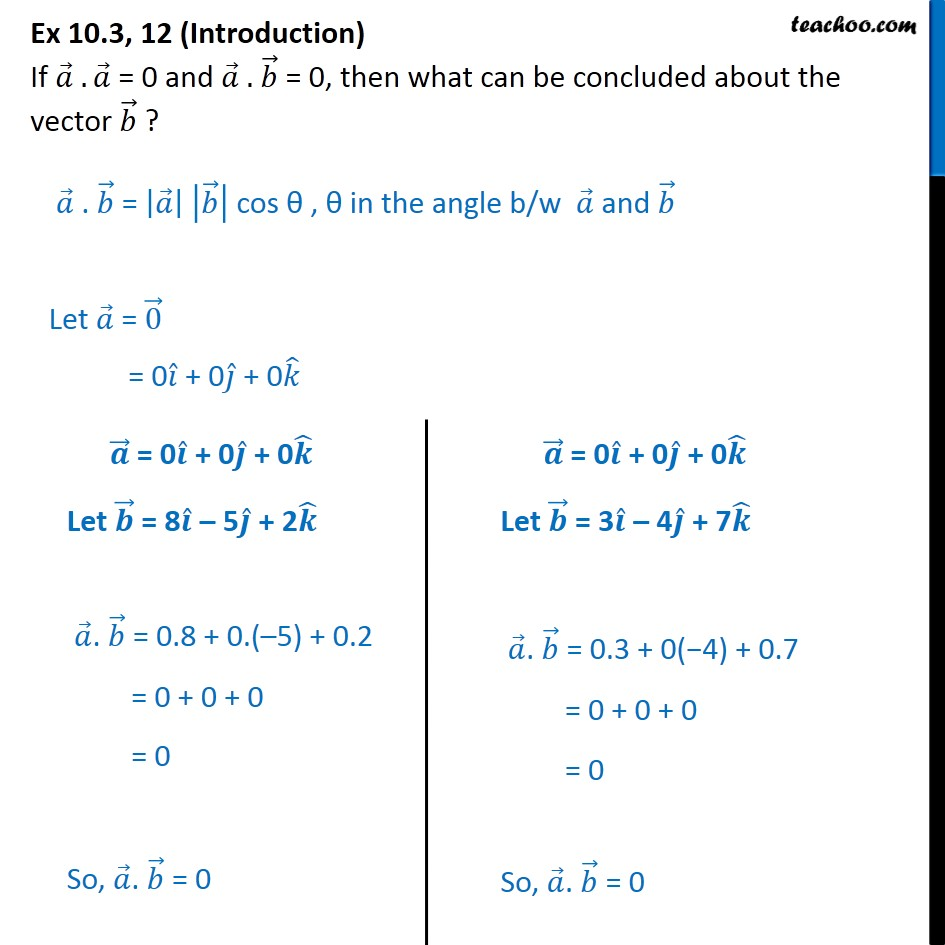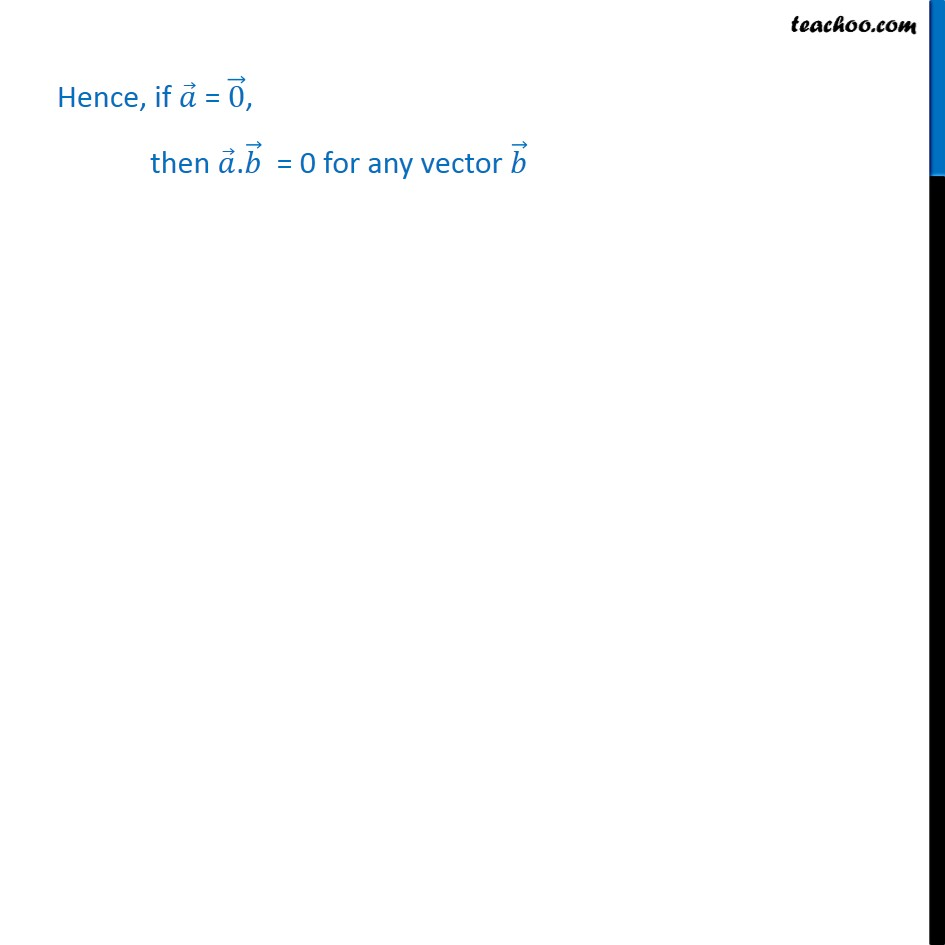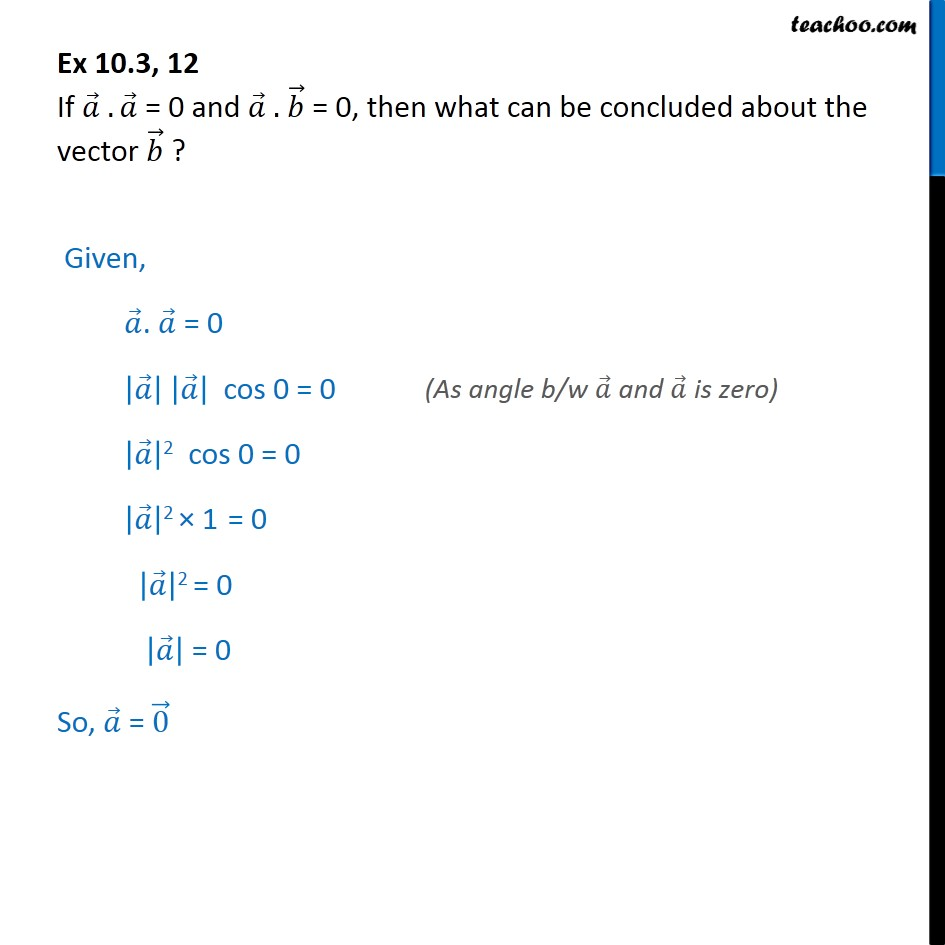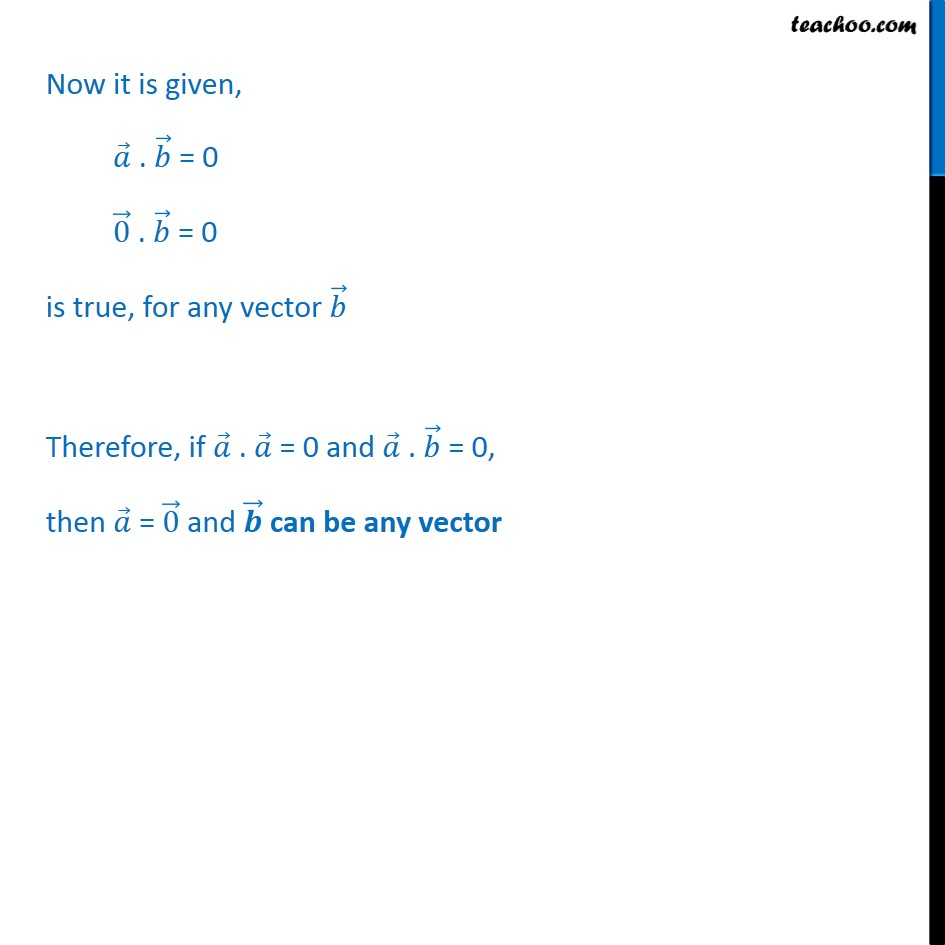1. Chapter 10 Class 12 Vector Algebra
2. Serial order wise
3. Ex 10.3

Transcript

Ex 10.3, 12 (Introduction) If 𝑎﷯ . 𝑎﷯ = 0 and 𝑎﷯ . 𝑏﷯ = 0, then what can be concluded about the vector 𝑏﷯ ? 𝑎﷯ . 𝑏﷯ = 𝑎﷯﷯ 𝑏﷯﷯ cos θ , θ in the angle b/w 𝑎﷯ and 𝑏﷯ Let 𝑎﷯ = 0﷯ = 0 𝑖﷯ + 0 𝑗﷯ + 0 𝑘﷯ Ex 10.3, 12 If 𝑎﷯ . 𝑎﷯ = 0 and 𝑎﷯ . 𝑏﷯ = 0, then what can be concluded about the vector 𝑏﷯ ? Given, 𝑎﷯. 𝑎﷯ = 0 𝑎﷯﷯ 𝑎﷯﷯ cos 0 = 0 𝑎﷯﷯2 cos 0 = 0 𝑎﷯﷯2 × 1 = 0 𝑎﷯﷯2 = 0 𝑎﷯﷯ = 0 So, 𝑎﷯ = 0﷯ Now it is given, 𝑎﷯ . 𝑏﷯ = 0 0﷯ . 𝑏﷯ = 0 is true, for any vector 𝑏﷯ Therefore, if 𝑎﷯ . 𝑎﷯ = 0 and 𝑎﷯ . 𝑏﷯ = 0, then 𝑎﷯ = 0﷯ and 𝒃﷯ can be any vector

Ex 10.3